### Home > CALC > Chapter 1 > Lesson 1.5.1 > Problem1-196

1-196.
1. Given the tables below, 1-196 HW eTool (Desmos). Homework Help ✎

 x f(x) −2 −1 0 1 2 3 10 100 −11 −8 −5 −2 1 4 25 295

 x g(x) −3 −2 −1 0 1 2 3 12 −5 0 3 4 3 0 −5 −140

 x h(x) −2π −π 0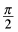π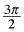2π 12π 2 −2 2 0 −2 0 2 2

1. Find possible functions for f(x), g(x), and h(x).

2. Evaluate:

1. f(g(h(π)))

2. h(g−1 (4))

3. f −1(h(π))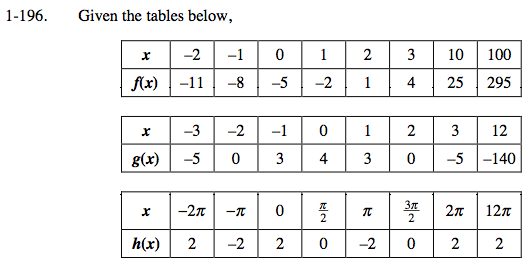Use the eTool below to explore.
Click the link at right for the full version of the eTool: Calc 1-196 HW eTool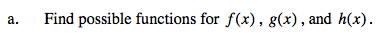f(x) looks linear.
h(x) looks trigonometric.
Find a transformation that works for each of them.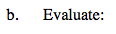These composite functions can be evaluated with the table.You do not need to use the equations from part (a).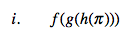h(π) = −(2)
g(−2) = 0
f(0) = −5
so f(g(h(π))) = −5g −1(4)
Translation: On the g(x) table, what x-value has a y-value of 4? Use the table... do NOT find the inverse function... that would be a waste of time!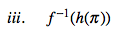Refer to hint in part (ii).

f −1(h(π)) = 1